# Sample Data: Amacrine Cells

Locations of amacrine cells (inhibitory interneurons in the retina) annotated with on/off marks

## Details

Locations of amacrine cells in the observation region Rectangle[{0, 0}, {1.6012085, 1}]*662 microns, annotated with on/off marks.

## Examples

### Basic Examples (1)

 In:=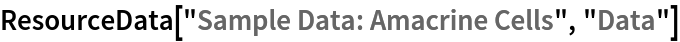Out=Summary of the spatial point data:

 In:=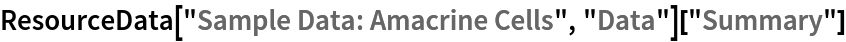Out=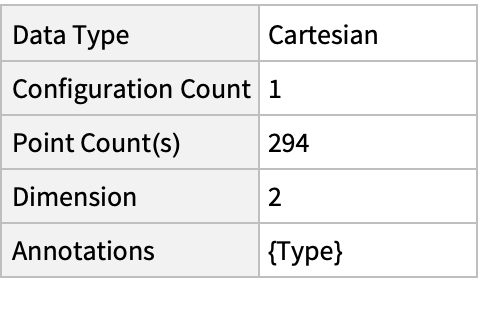### Visualizations (2)

Plot the spatial point data:

 In:=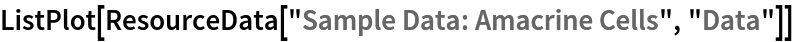Out=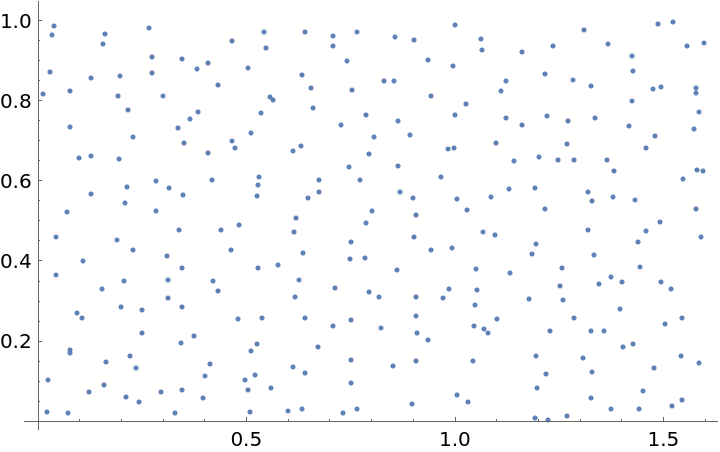Plot annotated locations:

 In:=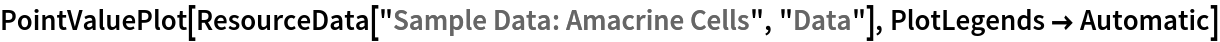Out=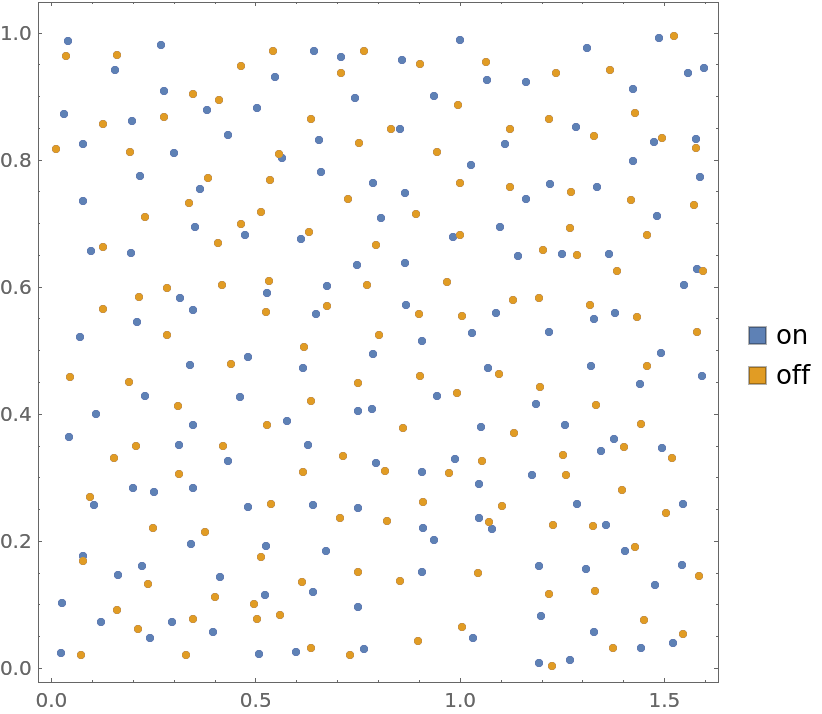### Analysis (4)

Compute probability of finding a point within given radius of an existing point - NearestNeighborG is the CDF of the nearest neighbor distribution:

 In:=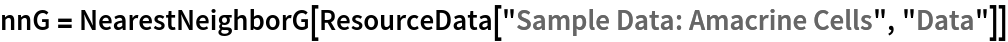Out=In:=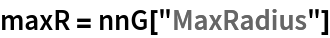Out=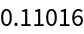In:=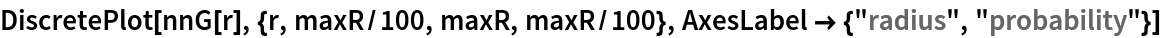Out=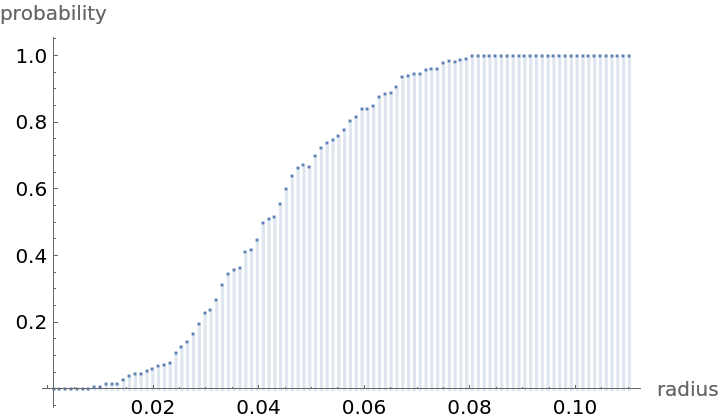Mean distance between a typical point and its nearest neighbor (for positive support distribution can be approximated via a Riemann sum of 1-CDF):

 In:=In:=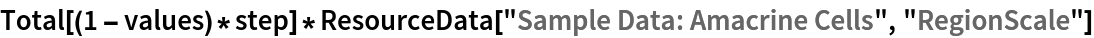Out=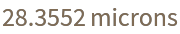Test for complete spacial randomness:

 In:=Out=Fit a Poisson point process to data:

 In:=Out=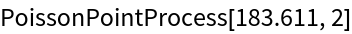Gosia Konwerska, "Sample Data: Amacrine Cells" from the Wolfram Data Repository (2021)